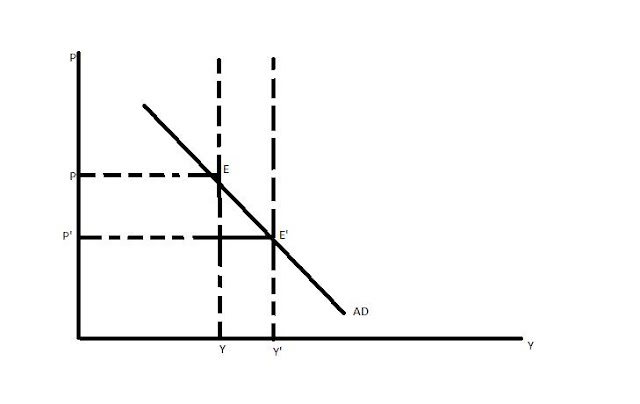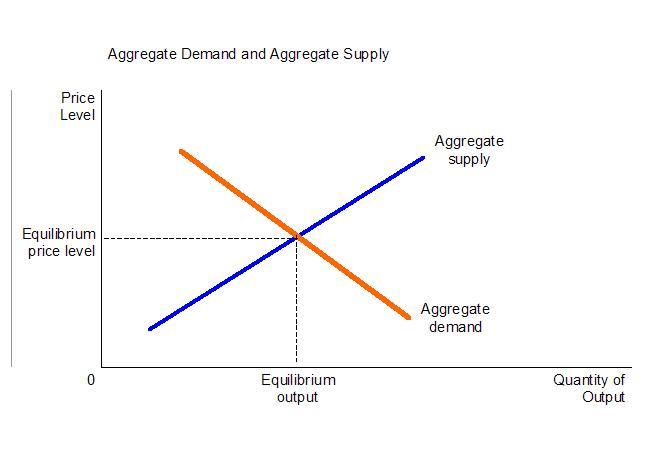# Aggregate Demand, Aggregate Supply and Equilibrium

## Aggregate Demand:

Aggregate Demand is the total quantity of all goods and services demanded in an economy. Over a long period of time, Aggregate Demand becomes the Gross Domestic Product of an economy. Aggregate Demand can be broken down into consumer goods, capital goods, exports, imports, and government spending. The aggregate demand curve is downward sloping, hence showing a negative relation between prices and quantity of the goods.AD = C + I + G +(X – M)
Where:
Aggregate Demand
C = Consumer spending on goods and services
I = Investment expenditure of private and corporate firms
G = Government expenditure
X – M = Net exports (exports minus imports)

### Factors affecting Aggregate Demand and Shifts in Aggregate Supply Curve:

• Changes in Interest Rates: If Interest rates increases, then consumers and business firms will get finance at high rates, hence their expenditure will decrease, resulting in a decrease in aggregate demand (aggregate demand curve will shift downwards). While due to a decrease in interest rates, consumers and business firms will get finance at lower rates, resulting in an increase in spending, and hence, aggregate demand will increase (aggregate demand curve will shift upwards).
• Income and Wealth: If the income of the household increases, then they will have more money to spend, so aggregate demand will increase (the aggregate demand curve will shift upwards). Conversely, if the income of the household decreases, then they will have less amount of money for spending, so aggregate demand will decrease (aggregate demand curve will shift downwards).
• Currency Exchange Rate Changes: If the value of the currency of a country rises or falls, then foreign goods will become more or less expensive, hence aggregate demand will increase (aggregate demand curve will shift downwards) or decrease (aggregate demand curve will shift downwards).

## Aggregate Supply:

Aggregate Supply is the total amount of the goods produced in an economy at a given price for a particular period. Aggregate Supply changes in the short-run due to the changes in the aggregate demand. The aggregate demand curve is upward sloping, as a supplier is willing to supply more at high prices and less at low prices.

### Factors affecting Aggregate Supply and Shifts in Aggregate Supply Curve:

There are various factors that affect the aggregate supply curves and curve shifts from its original position such as:
• technological advancements (aggregate supply curve will shift upwards
• Quantity and Quality of labor (due to increased efficiency of labor, the aggregate supply curve will shift upwards and increase in wages of labor will shift aggregate supply curve downwards as production cost will increase and hence production will decrease)
• taxes and subsidies (due to imposition of taxes and reduction of subsidies, the aggregate supply curve will shift downwards and due to relaxation of taxes and grant of subsidies, the aggregate supply curve will shift upwards),

## Inflation

### Equilibrium GDP and Prices:

• Equilibrium is obtained when aggregate demand equals aggregate supply. At the level of price, where they become equal is the equilibrium price and the level of output is GDP.
• As can be seen in the below diagram, the price and output level where aggregate demand and aggregate supply curve intersects are equilibrium price level and equilibrium output level (GDP).